# NCERT Exemplar Solutions for Class 12 Maths Chapter 11 Three Dimensional Geometry

The right tool is always required for students to prepare well for their examinations. The NCERT Exemplar textbooks act as a tool in providing advanced knowledge on concepts of Classes 6 to 12 for CBSE students. The NCERT Exemplar Solutions can be accessed by students to clear any doubts and also to solve the exercise problems. The solutions are created by individual subject experts at BYJU’S, according to the latest CBSE guidelines. This ensures students score high marks in their examinations.

Chapter 11 of the NCERT Exemplar Solutions for Class 12 Mathematics is Three Dimensional Geometry. The chapter has exemplar questions based on the direction cosines and ratios of a line, the relation between the direction cosines of a line, the direction cosines of a line passing through two points, the angle between two lines, the equation of a plane perpendicular to a given vector and passing via a given point equation of a plane in normal form coplanarity of two lines, etc. To help students with their exam preparations, the solutions PDF of NCERT Exemplar Solutions for Class 12 Maths Chapter 11 Three Dimensional Geometry is available in the link given below.

## Download the PDF of NCERT Exemplar Solutions for Class 12 Maths Chapter 11 Three Dimensional Geometry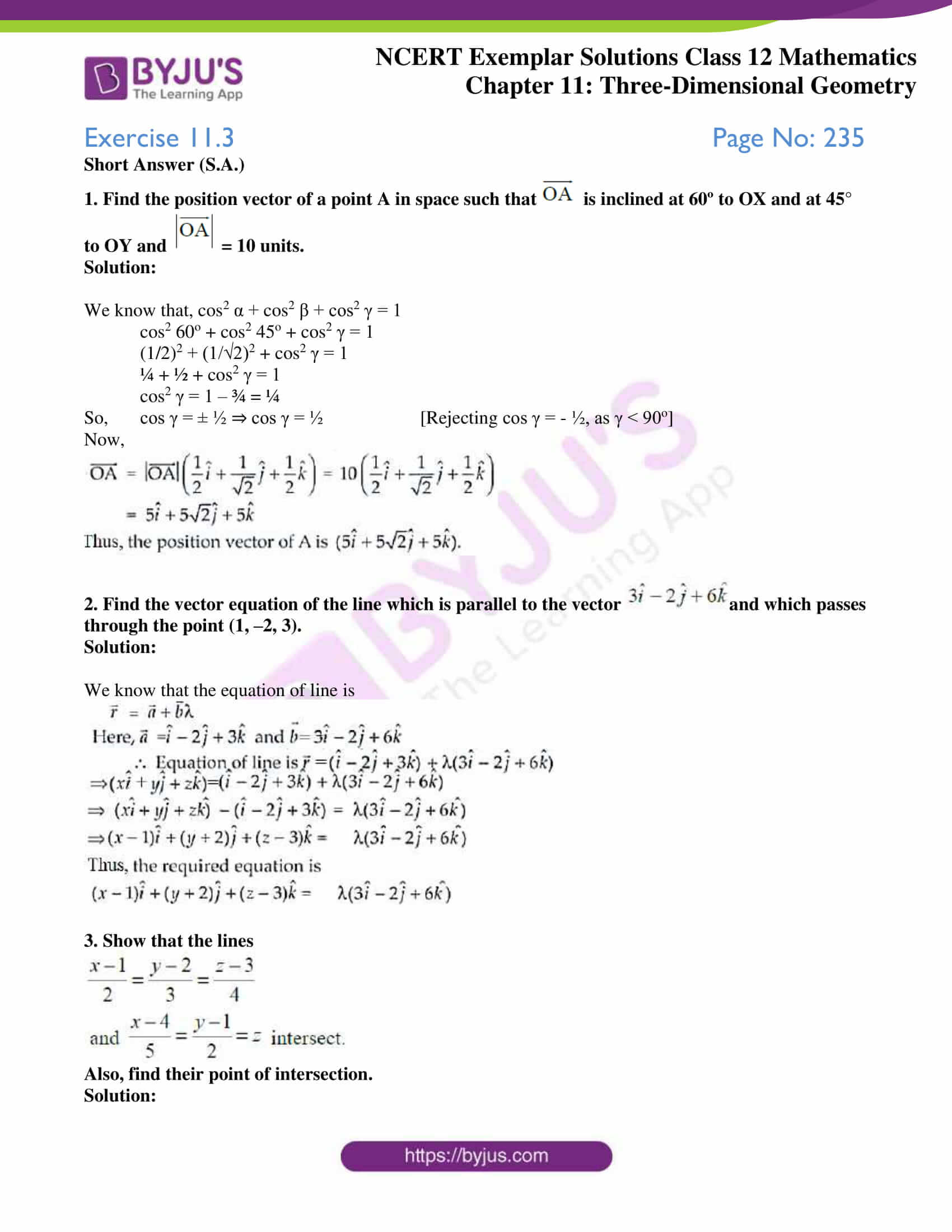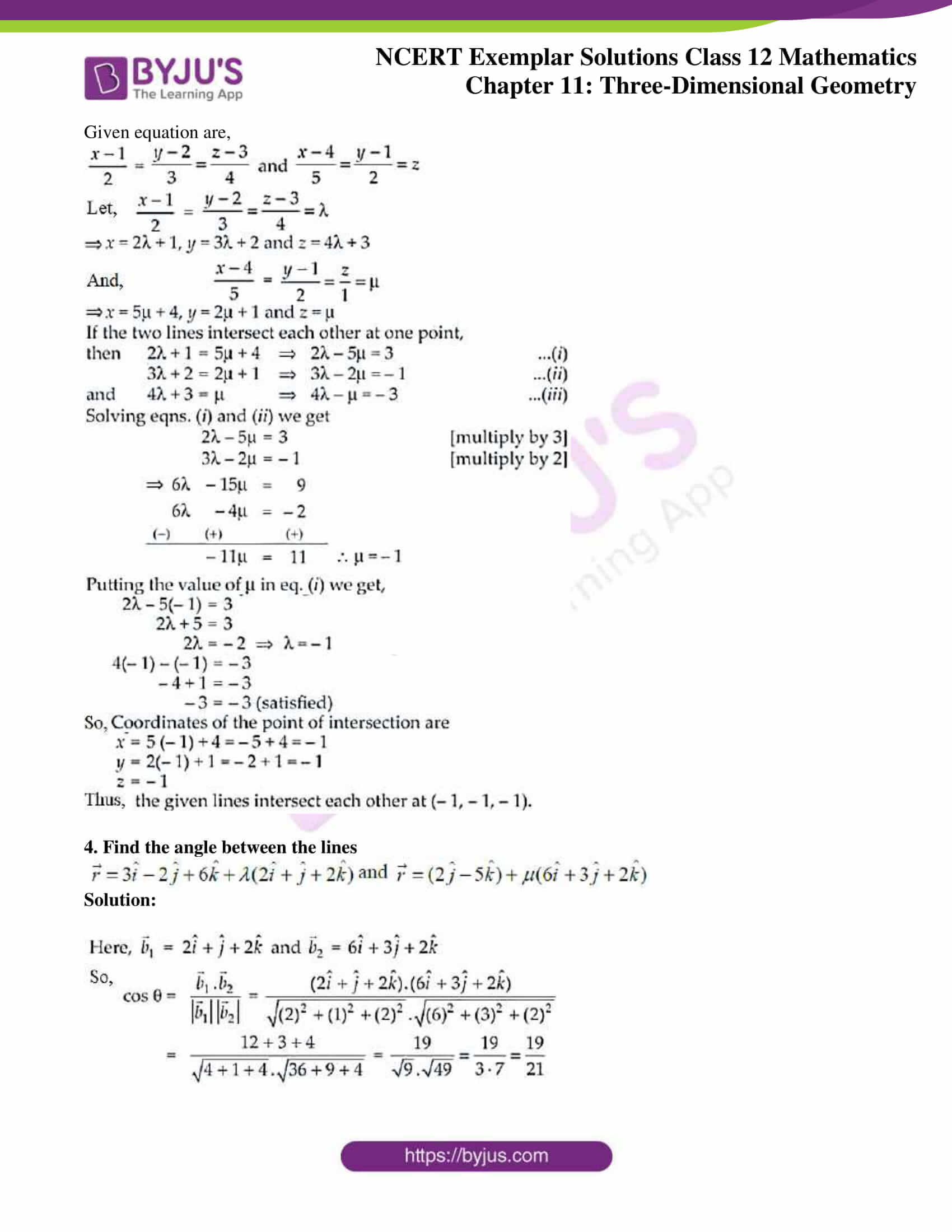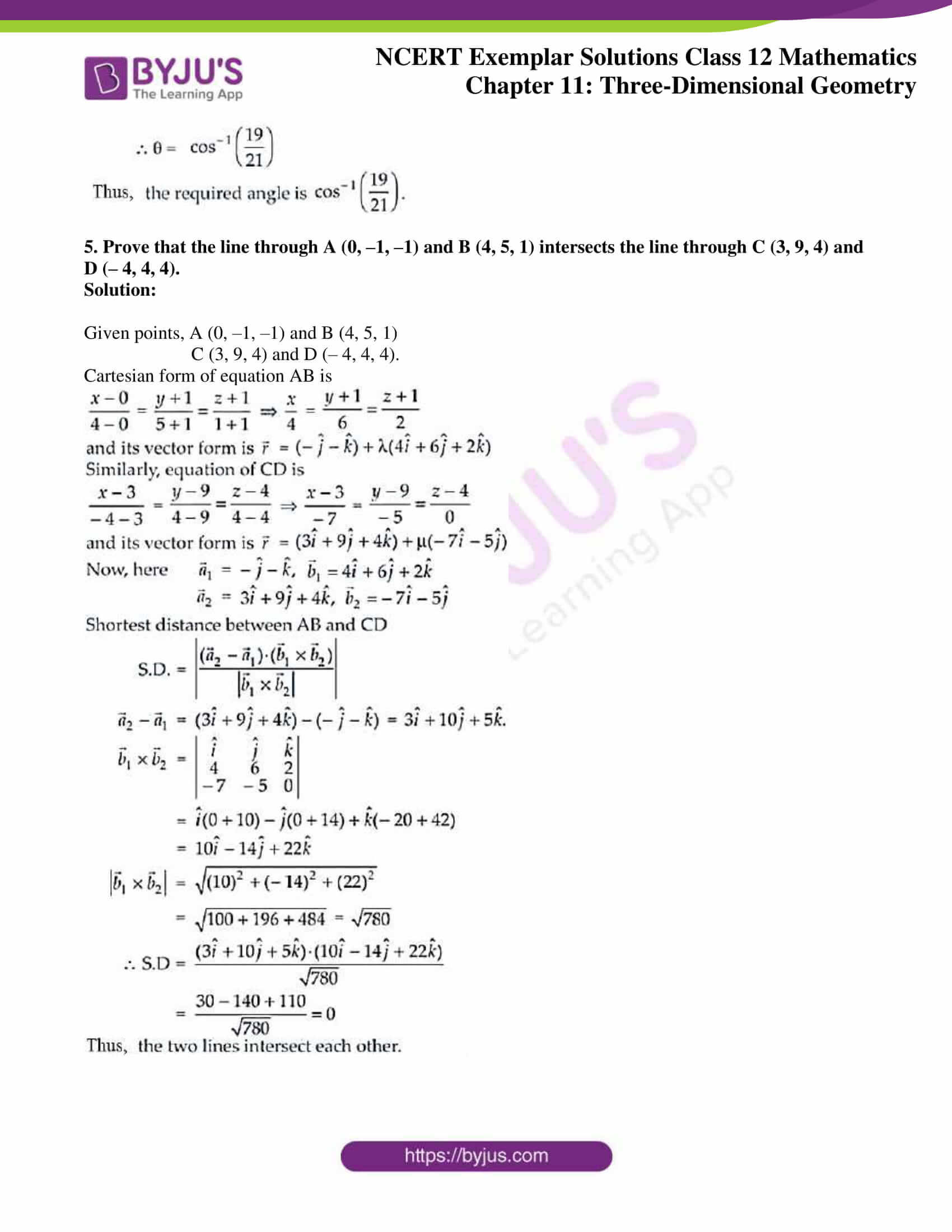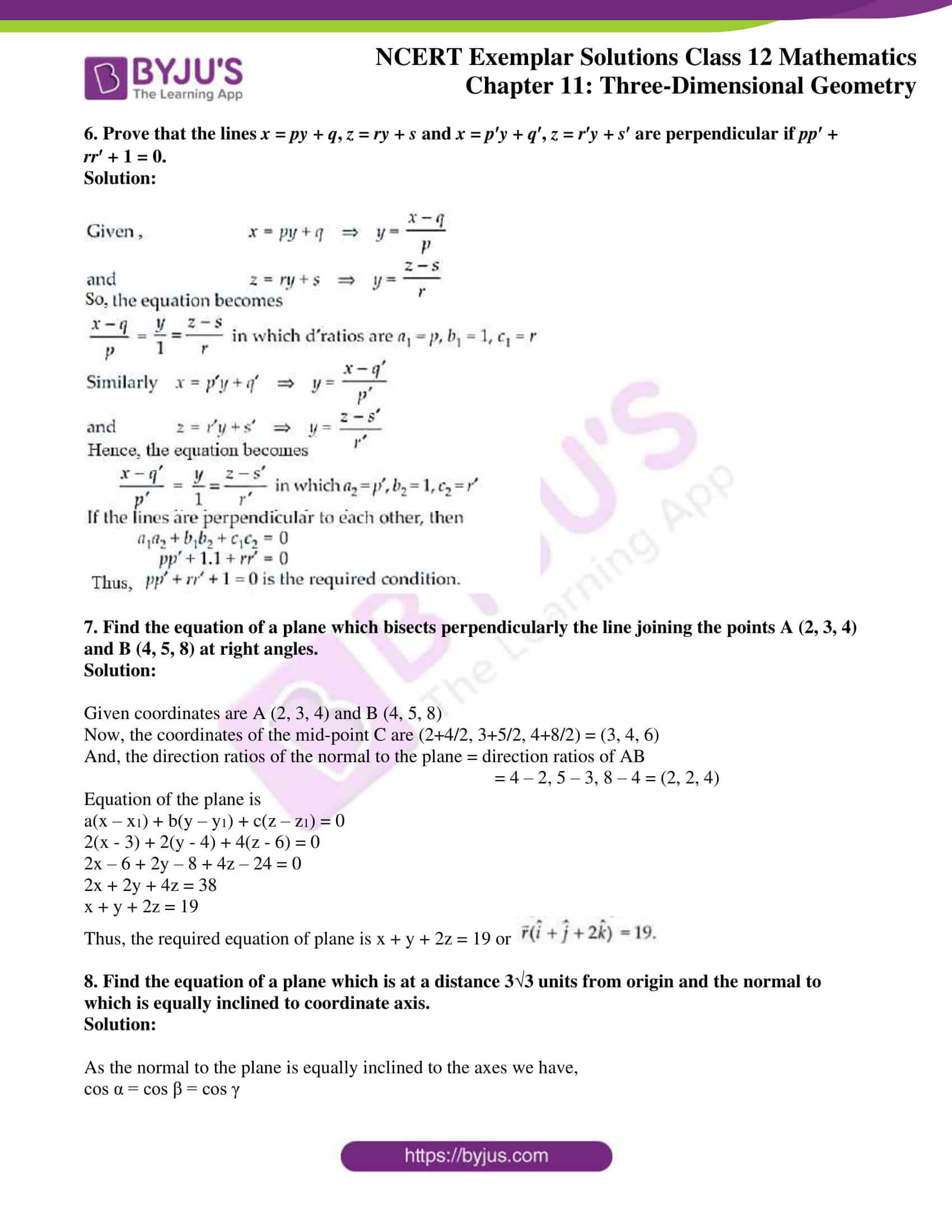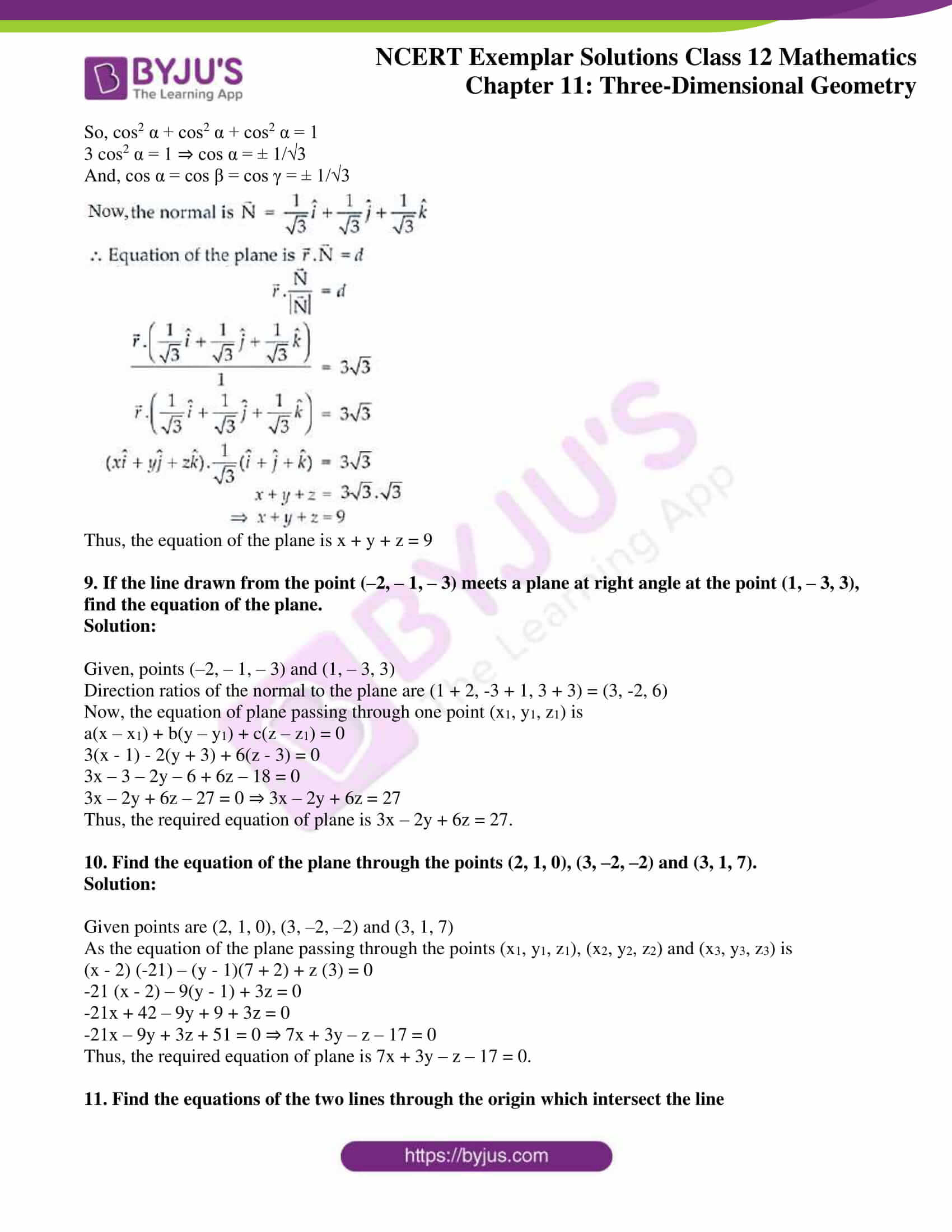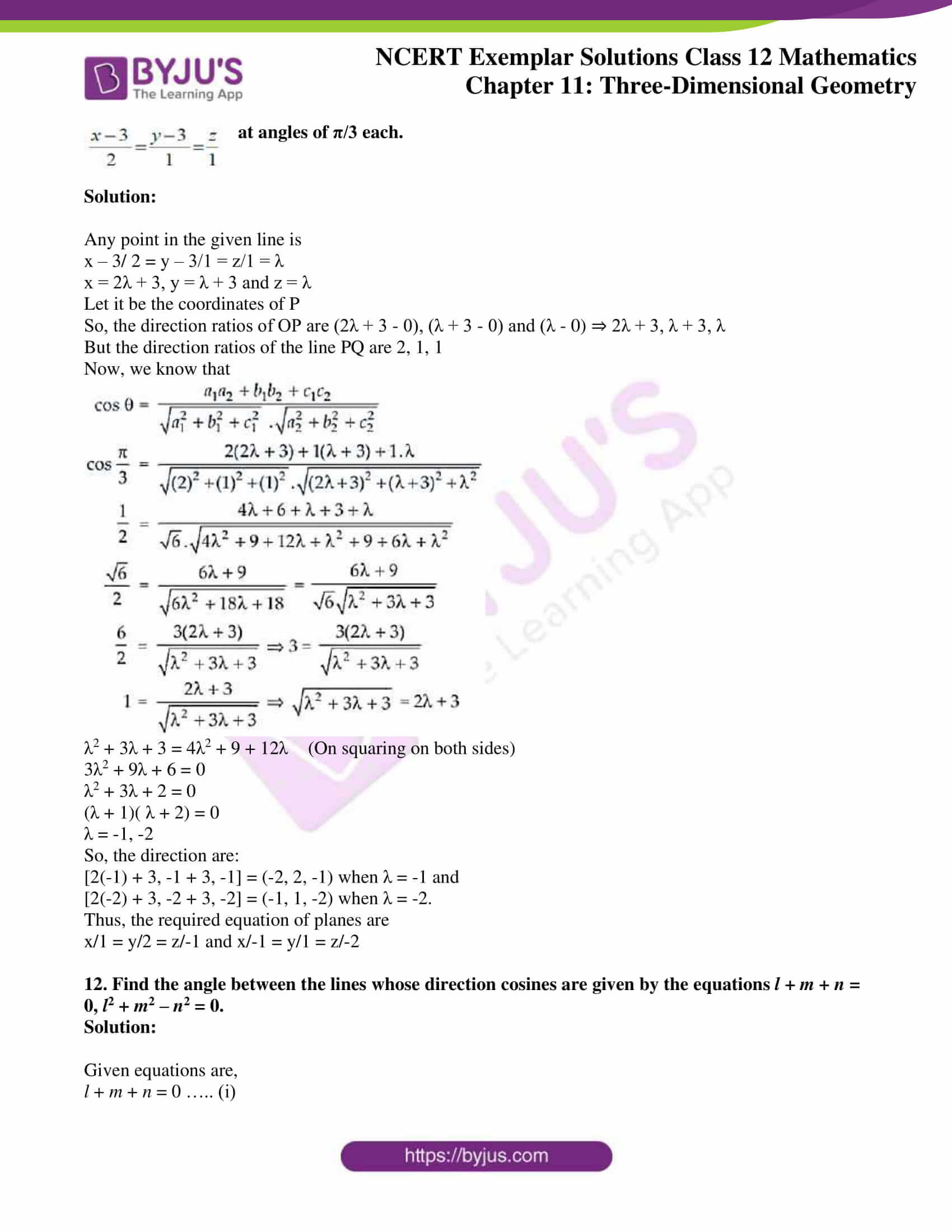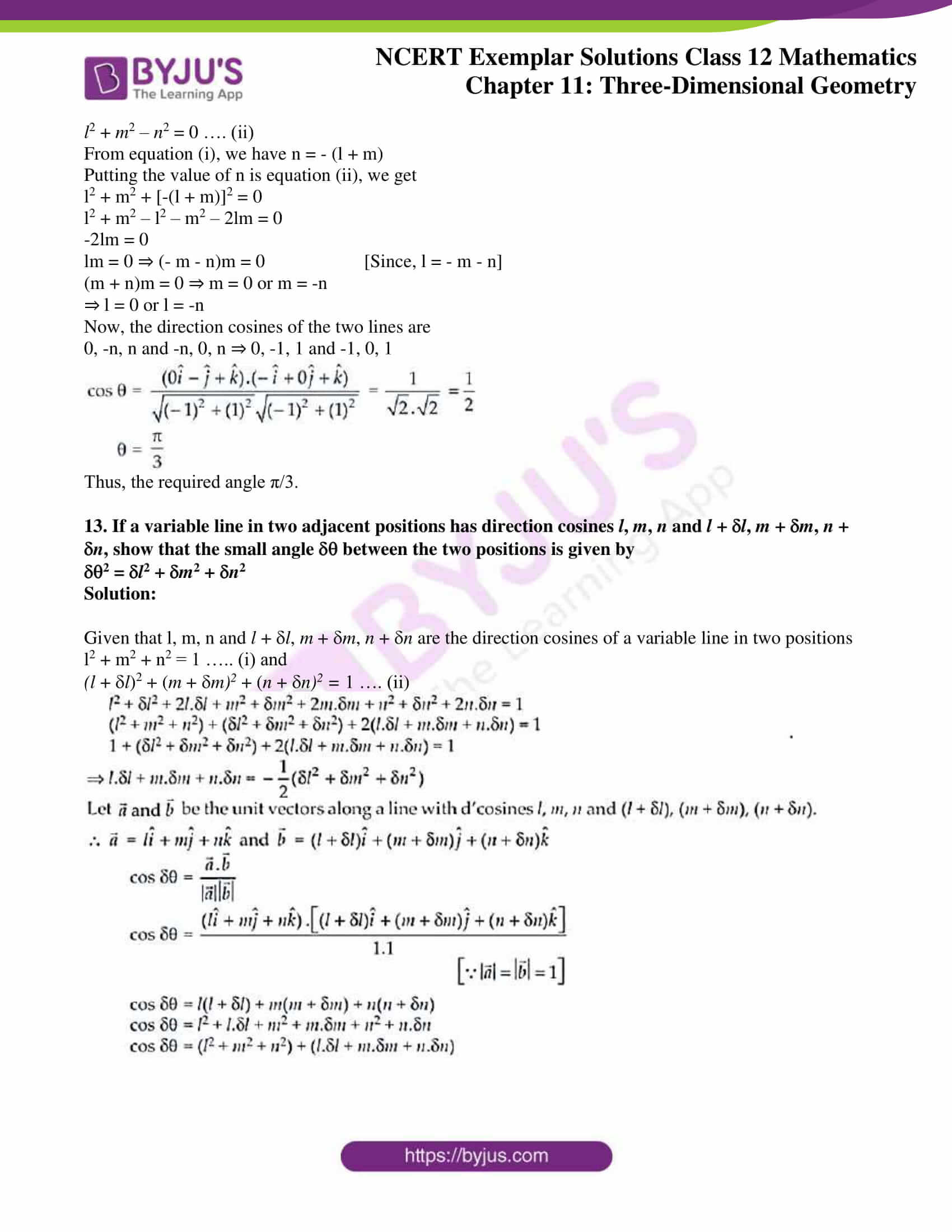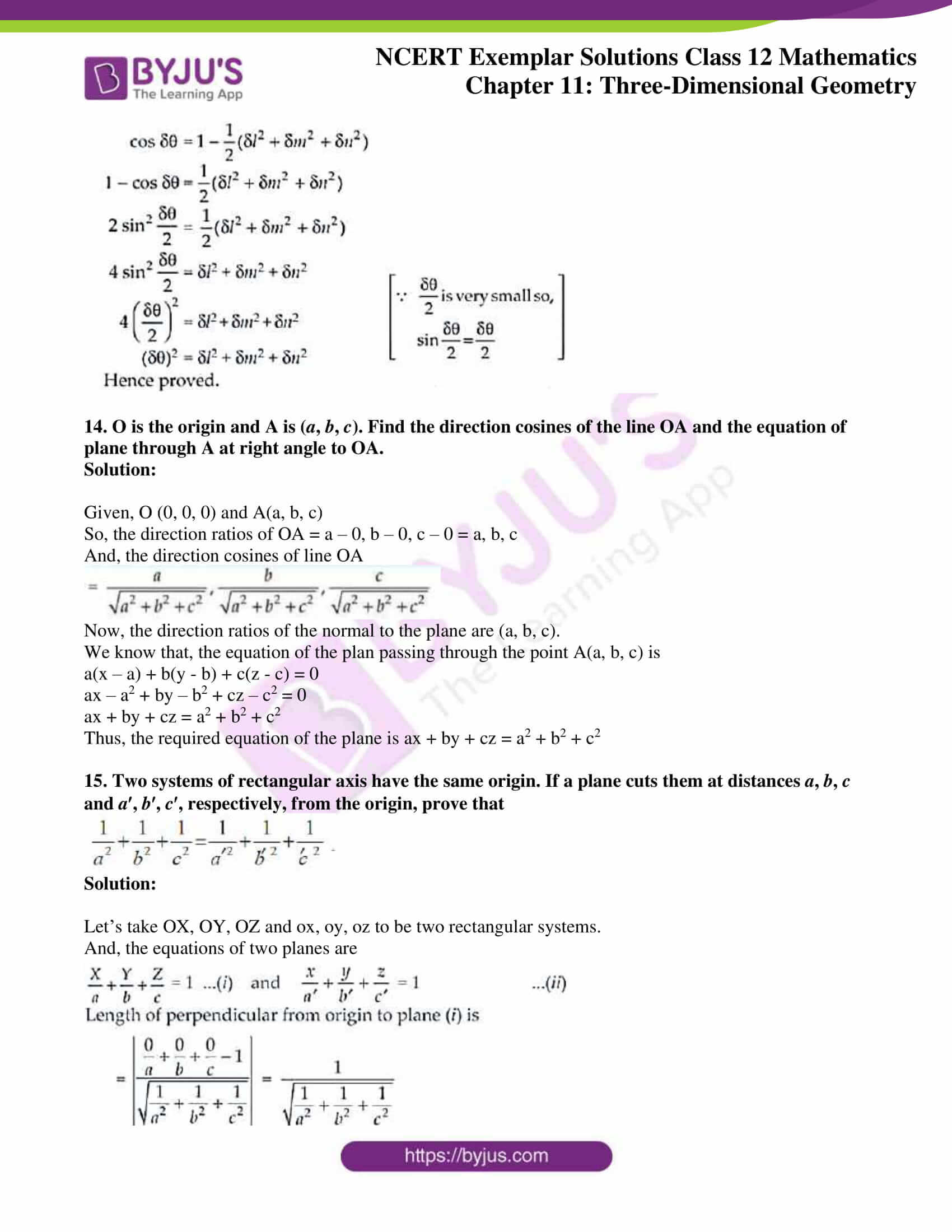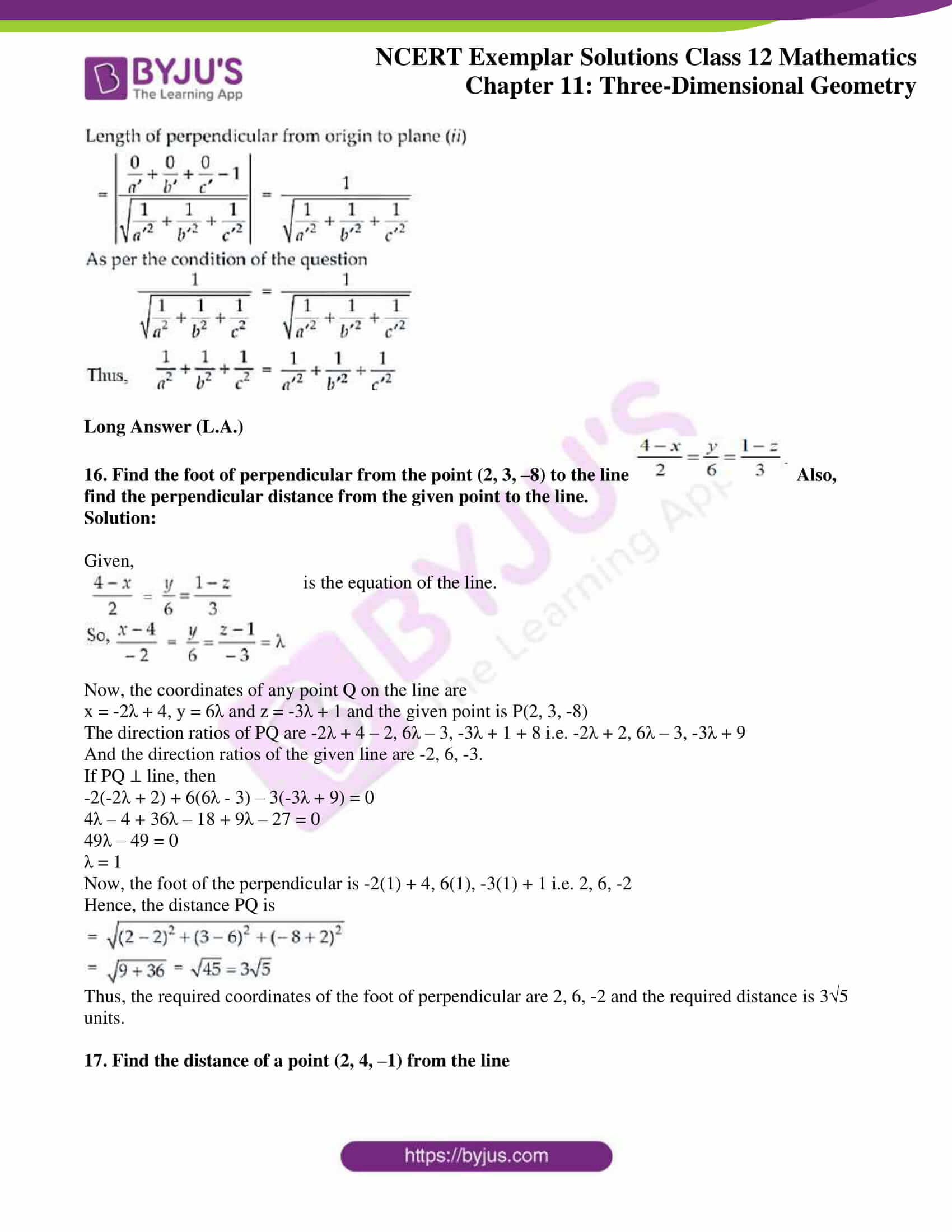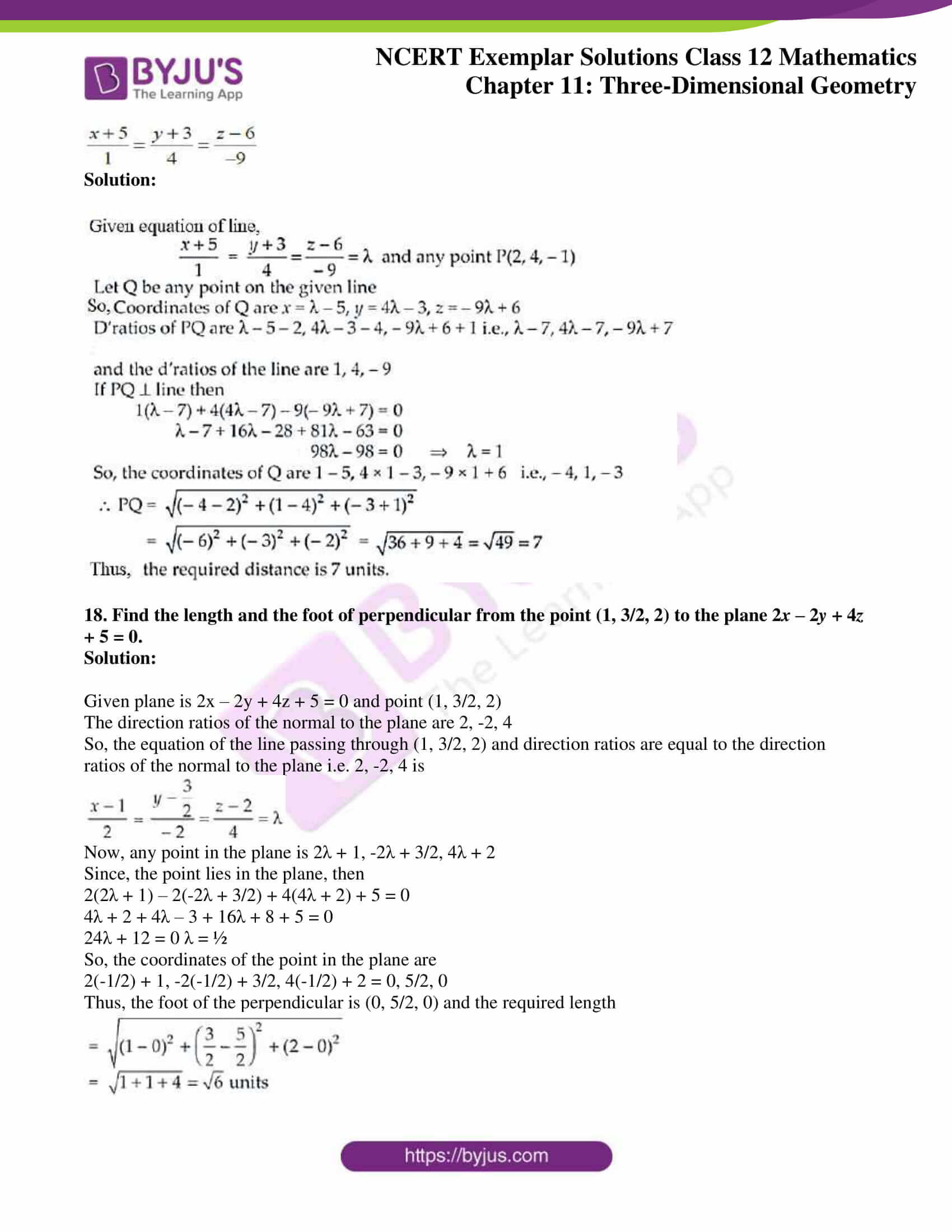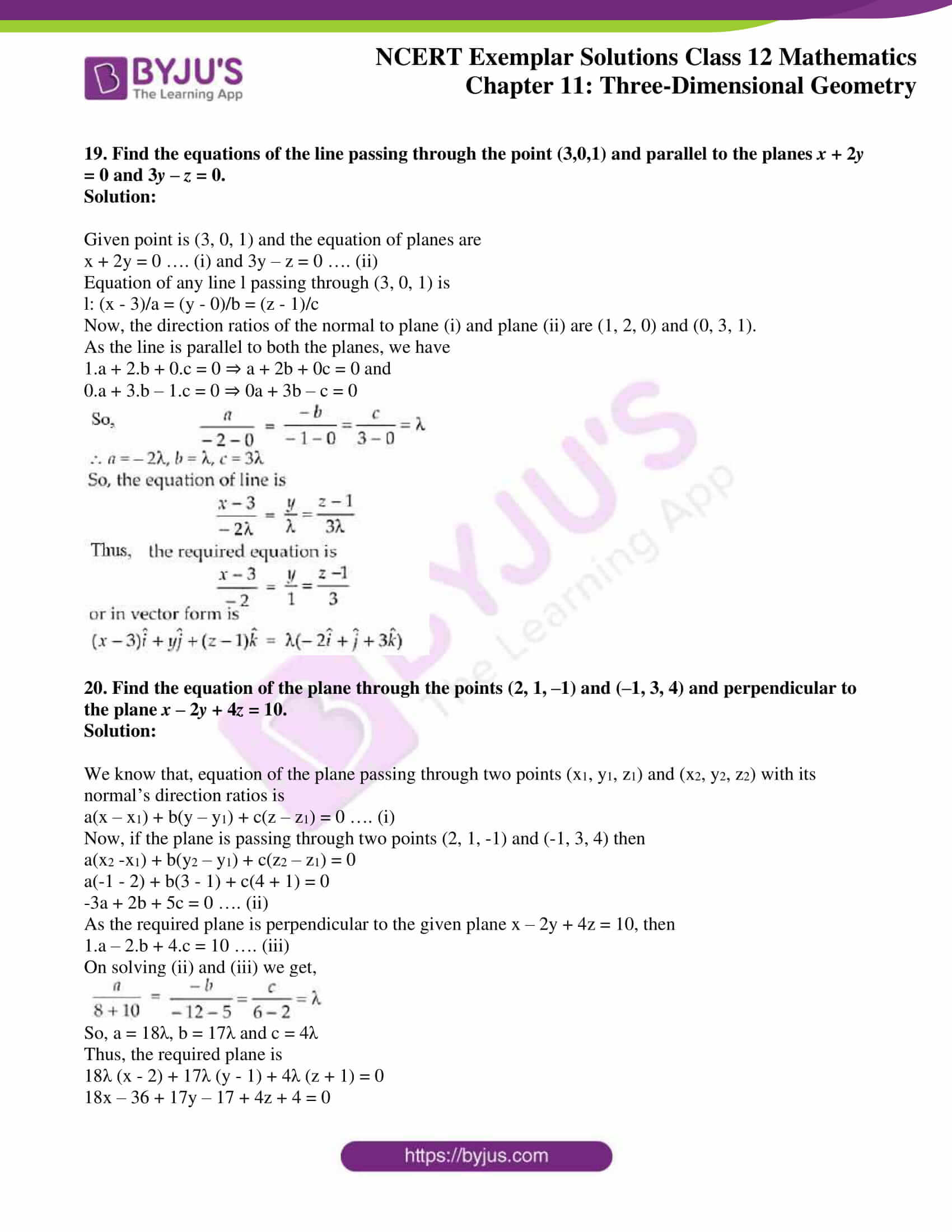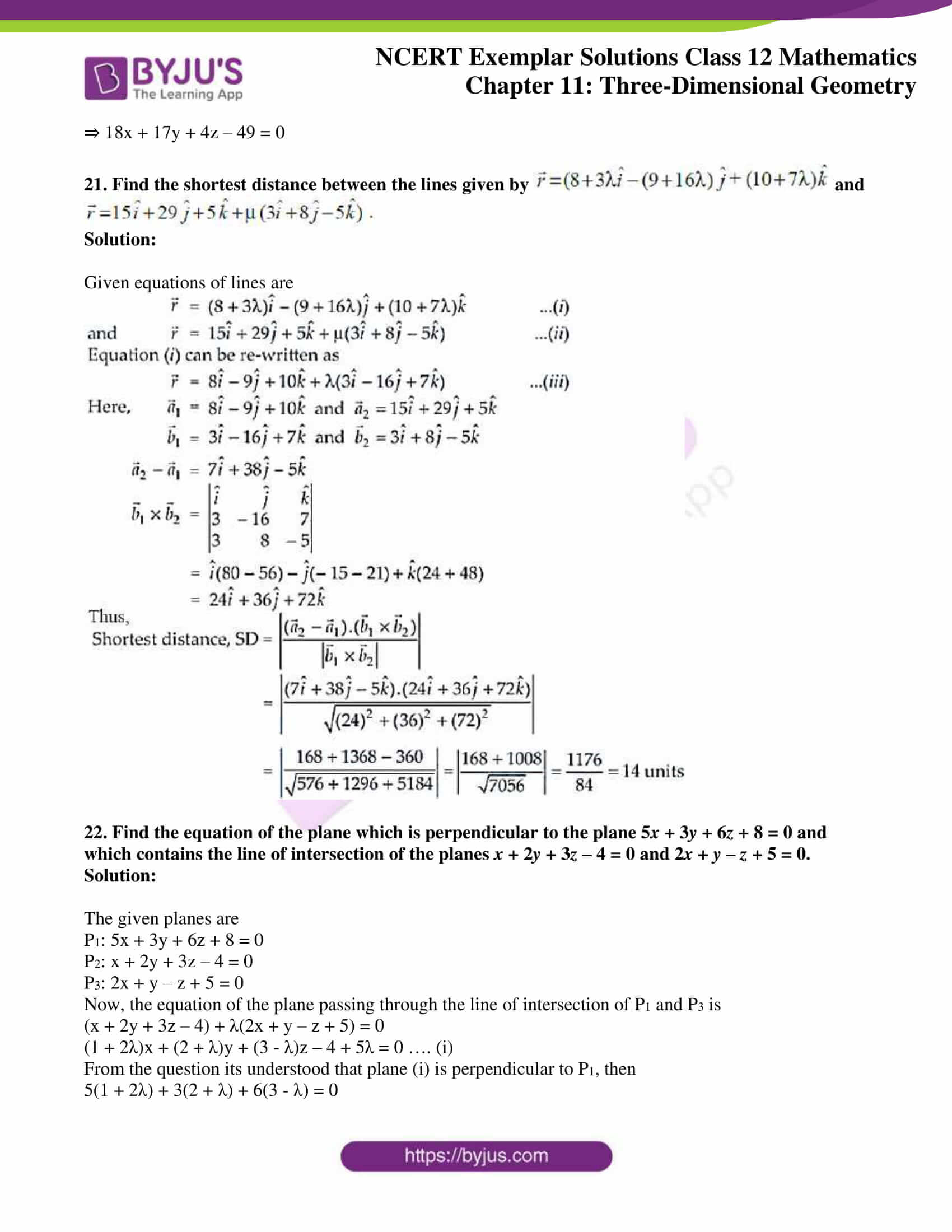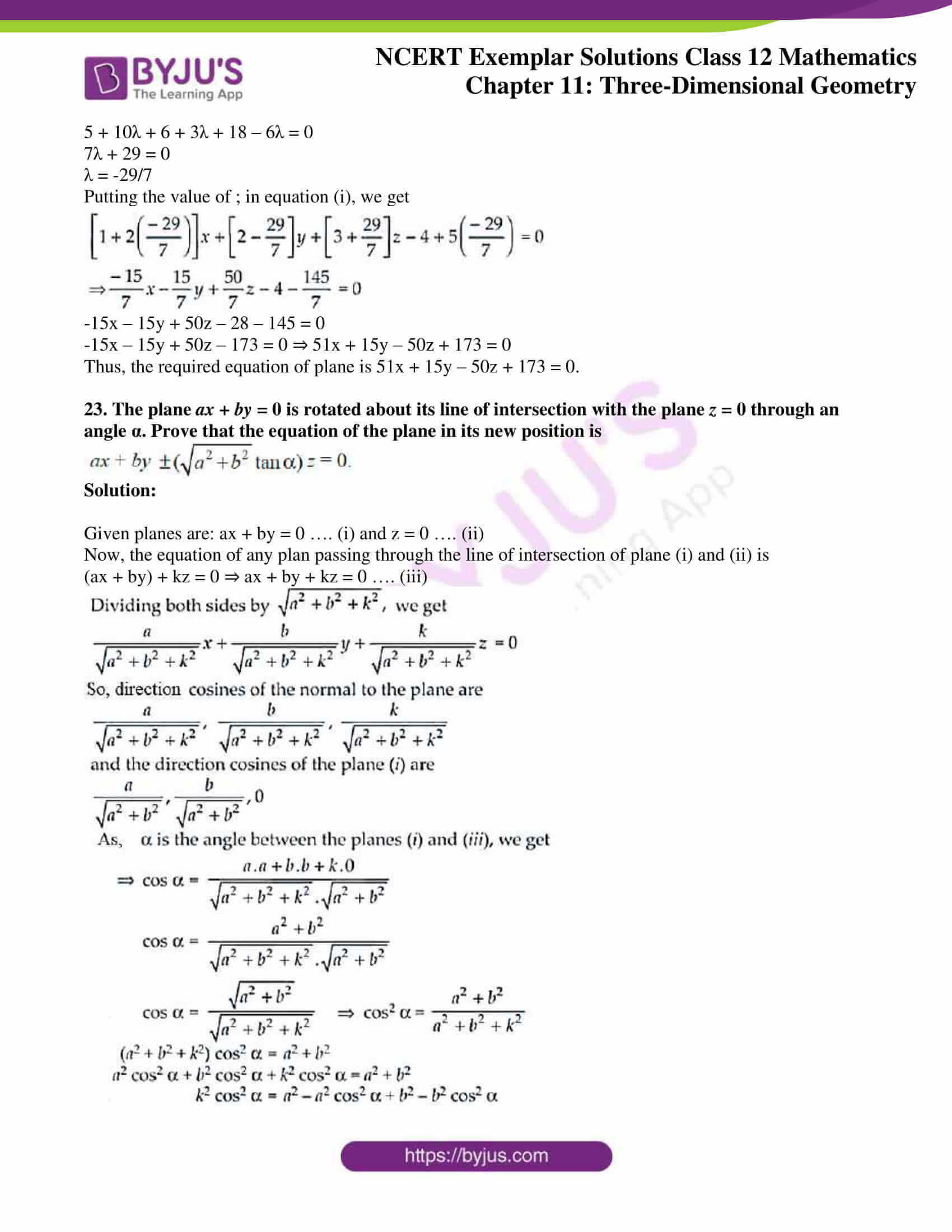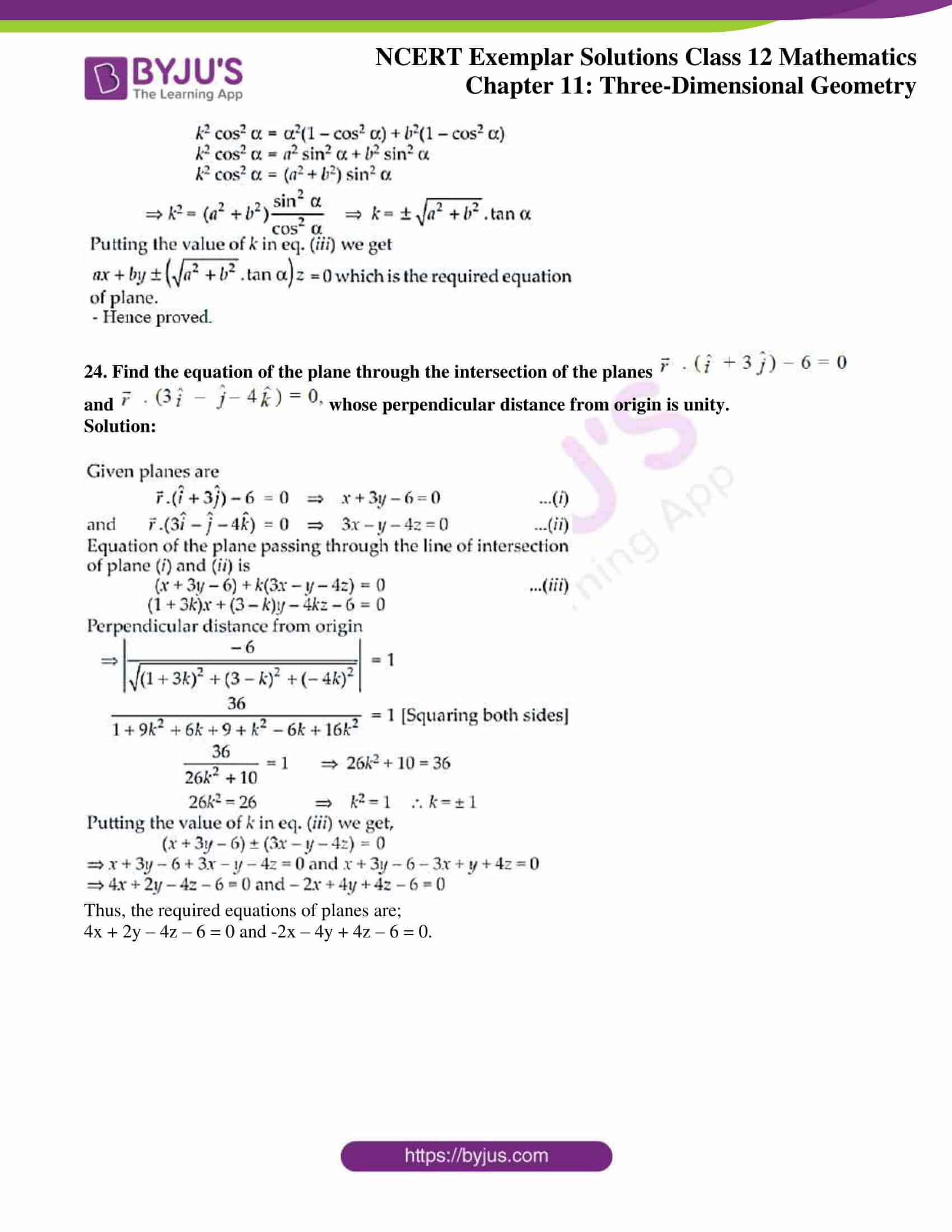### Access Answers to NCERT Exemplar Solutions for Class 12 Maths Chapter 11 Three Dimensional Geometry

Exercise 11.3 Page No: 235

1. Find the position vector of a point A in space such that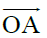is inclined at 60º to OX and at 45° to OY and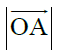= 10 units.

Solution:

We know that, cos2 α + cos2 β + cos2 γ = 1

cos2 60o + cos2 45o + cos2 γ = 1

(1/2)2 + (1/√2)2 + cos2 γ = 1

¼ + ½ + cos2 γ = 1

cos2 γ = 1 – ¾ = ¼

So, cos γ = ± ½ ⇒ cos γ = ½ [Rejecting cos γ = – ½, as γ < 90o]

Now,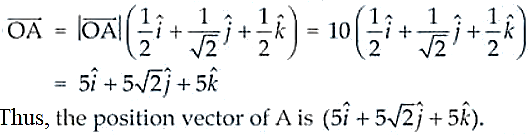2. Find the vector equation of the line which is parallel to the vector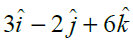and which passes through the point (1, –2, 3).

Solution:

We know that the equation of line is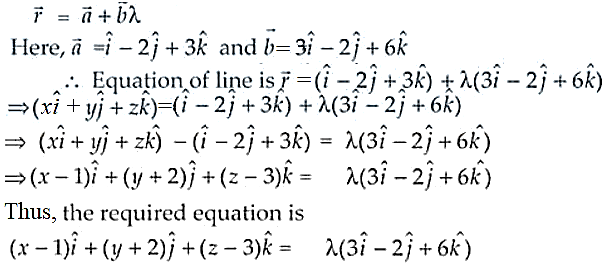3. Show that the lines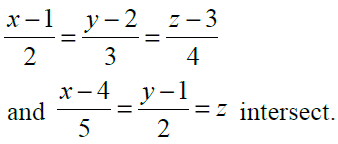Also, find their point of intersection.

Solution:

Given equation are,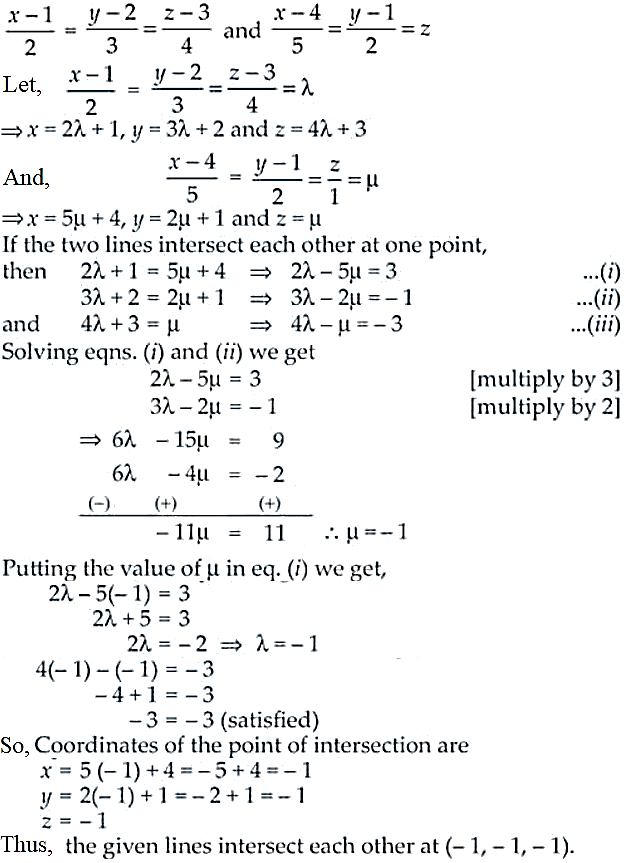4. Find the angle between the lines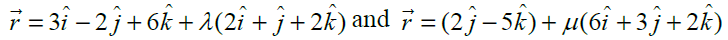Solution: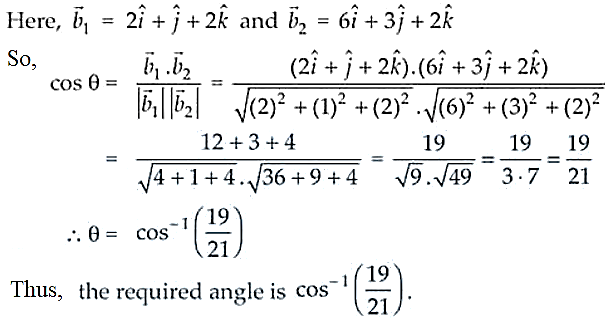5. Prove that the line through A (0, –1, –1) and B (4, 5, 1) intersects the line through C (3, 9, 4) and D (– 4, 4, 4).

Solution:

Given points, A (0, –1, –1) and B (4, 5, 1)

C (3, 9, 4) and D (– 4, 4, 4).

Cartesian form of equation AB is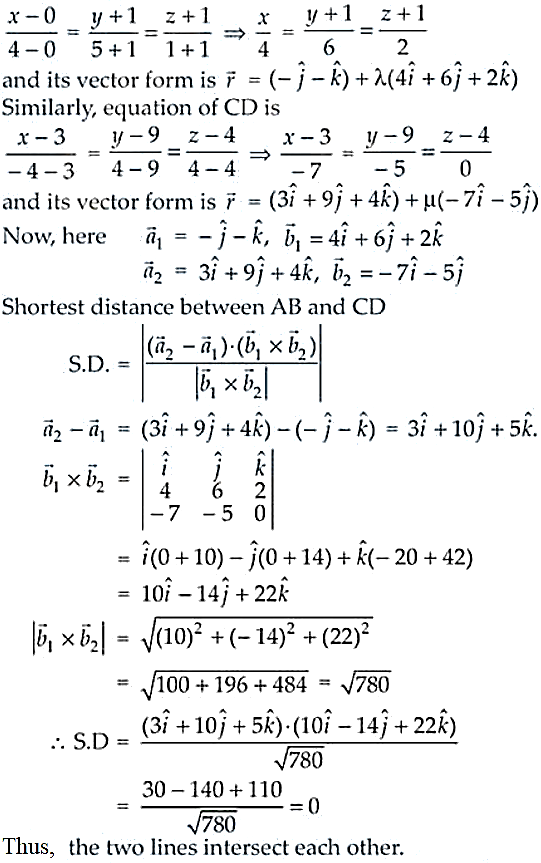6. Prove that the lines x = py + q, z = ry + s and x = p¢y + q¢, z = r¢y + s¢ are perpendicular if pp¢ + rr¢ + 1 = 0.

Solution: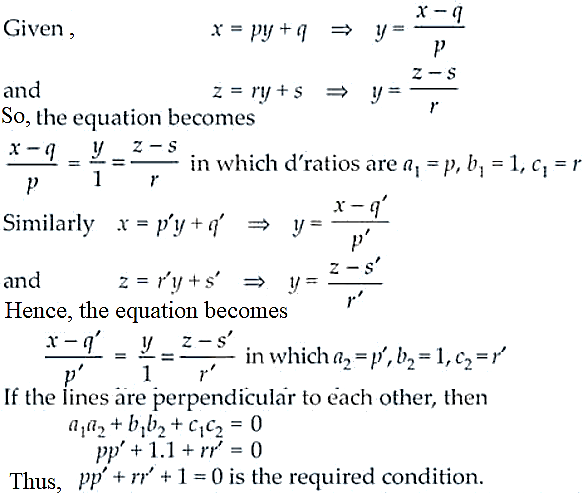7. Find the equation of a plane which bisects perpendicularly the line joining the points A (2, 3, 4) and B (4, 5, 8) at right angles.

Solution:

Given coordinates are A (2, 3, 4) and B (4, 5, 8)

Now, the coordinates of the mid-point C are (2+4/2, 3+5/2, 4+8/2) = (3, 4, 6)

And, the direction ratios of the normal to the plane = direction ratios of AB

= 4 – 2, 5 – 3, 8 – 4 = (2, 2, 4)

Equation of the plane is

a(x – x1) + b(y – y1) + c(z – z1) = 0

2(x – 3) + 2(y – 4) + 4(z – 6) = 0

2x – 6 + 2y – 8 + 4z – 24 = 0

2x + 2y + 4z = 38

x + y + 2z = 19

Thus, the required equation of plane is x + y + 2z = 19 or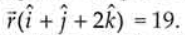8. Find the equation of a plane which is at a distance 3√3 units from origin and the normal to which is equally inclined to coordinate axis.

Solution:

As the normal to the plane is equally inclined to the axes we have,

cos α = cos β = cos γ

So, cos2 α + cos2 α + cos2 α = 1

3 cos2 α = 1 ⇒ cos α = ± 1/√3

And, cos α = cos β = cos γ = ± 1/√3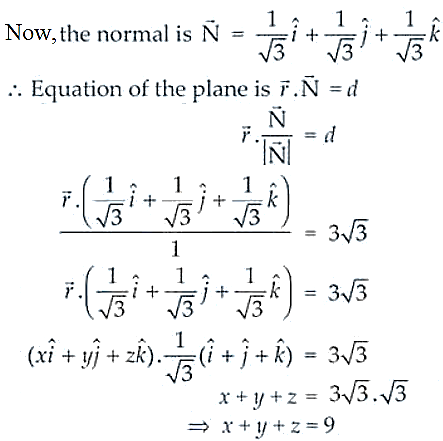Thus, the equation of the plane is x + y + z = 9

9. If the line drawn from the point (–2, – 1, – 3) meets a plane at right angle at the point (1, – 3, 3), find the equation of the plane.

Solution:

Given, points (–2, – 1, – 3) and (1, – 3, 3)

Direction ratios of the normal to the plane are (1 + 2, -3 + 1, 3 + 3) = (3, -2, 6)

Now, the equation of plane passing through one point (x1, y1, z1) is

a(x – x1) + b(y – y1) + c(z – z1) = 0

3(x – 1) – 2(y + 3) + 6(z – 3) = 0

3x – 3 – 2y – 6 + 6z – 18 = 0

3x – 2y + 6z – 27 = 0 ⇒ 3x – 2y + 6z = 27

Thus, the required equation of plane is 3x – 2y + 6z = 27.

10. Find the equation of the plane through the points (2, 1, 0), (3, –2, –2) and (3, 1, 7).

Solution:

Given points are (2, 1, 0), (3, –2, –2) and (3, 1, 7)

As the equation of the plane passing through the points (x1, y1, z1), (x2, y2, z2) and (x3, y3, z3) is

(x – 2) (-21) – (y – 1)(7 + 2) + z (3) = 0

-21 (x – 2) – 9(y – 1) + 3z = 0

-21x + 42 – 9y + 9 + 3z = 0

-21x – 9y + 3z + 51 = 0 ⇒ 7x + 3y – z – 17 = 0

Thus, the required equation of plane is 7x + 3y – z – 17 = 0.

11. Find the equations of the two lines through the origin which intersect the line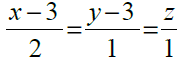at angles of π/3 each.

Solution:

Any point in the given line is

x – 3/ 2 = y – 3/1 = z/1 = λ

x = 2λ + 3, y = λ + 3 and z = λ

Let it be the coordinates of P

So, the direction ratios of OP are (2λ + 3 – 0), (λ + 3 – 0) and (λ – 0) ⇒ 2λ + 3, λ + 3, λ

But the direction ratios of the line PQ are 2, 1, 1

Now, we know that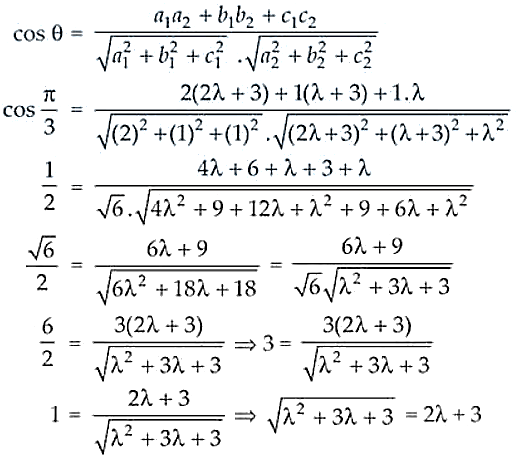λ2 + 3λ + 3 = 4λ2 + 9 + 12λ (On squaring on both sides)

2 + 9λ + 6 = 0

λ2 + 3λ + 2 = 0

(λ + 1)( λ + 2) = 0

λ = -1, -2

So, the direction are:

[2(-1) + 3, -1 + 3, -1] = (-2, 2, -1) when λ = -1 and

[2(-2) + 3, -2 + 3, -2] = (-1, 1, -2) when λ = -2.

Thus, the required equation of planes are

x/1 = y/2 = z/-1 and x/-1 = y/1 = z/-2

12. Find the angle between the lines whose direction cosines are given by the equations l + m + n = 0, l2 + m2n2 = 0.

Solution:

Given equations are,

l + m + n = 0 ….. (i)

l2 + m2n2 = 0 …. (ii)

From equation (i), we have n = – (l + m)

Putting the value of n is equation (ii), we get

l2 + m2 + [-(l + m)]2 = 0

l2 + m2 – l2 – m2 – 2lm = 0

-2lm = 0

lm = 0 ⇒ (- m – n)m = 0 [Since, l = – m – n]

(m + n)m = 0 ⇒ m = 0 or m = -n

⇒ l = 0 or l = -n

Now, the direction cosines of the two lines are

0, -n, n and -n, 0, n ⇒ 0, -1, 1 and -1, 0, 1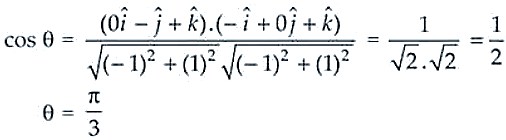Thus, the required angle π/3.

13. If a variable line in two adjacent positions has direction cosines l, m, n and l + dl, m + dm, n + dn, show that the small angle dq between the two positions is given by

dq2 = dl2 + dm2 + dn2

Solution:

Given that l, m, n and l + dl, m + dm, n + dn are the direction cosines of a variable line in two positions

l2 + m2 + n2 = 1 ….. (i) and

(l + dl)2 + (m + dm)2 + (n + dn)2 = 1 …. (ii)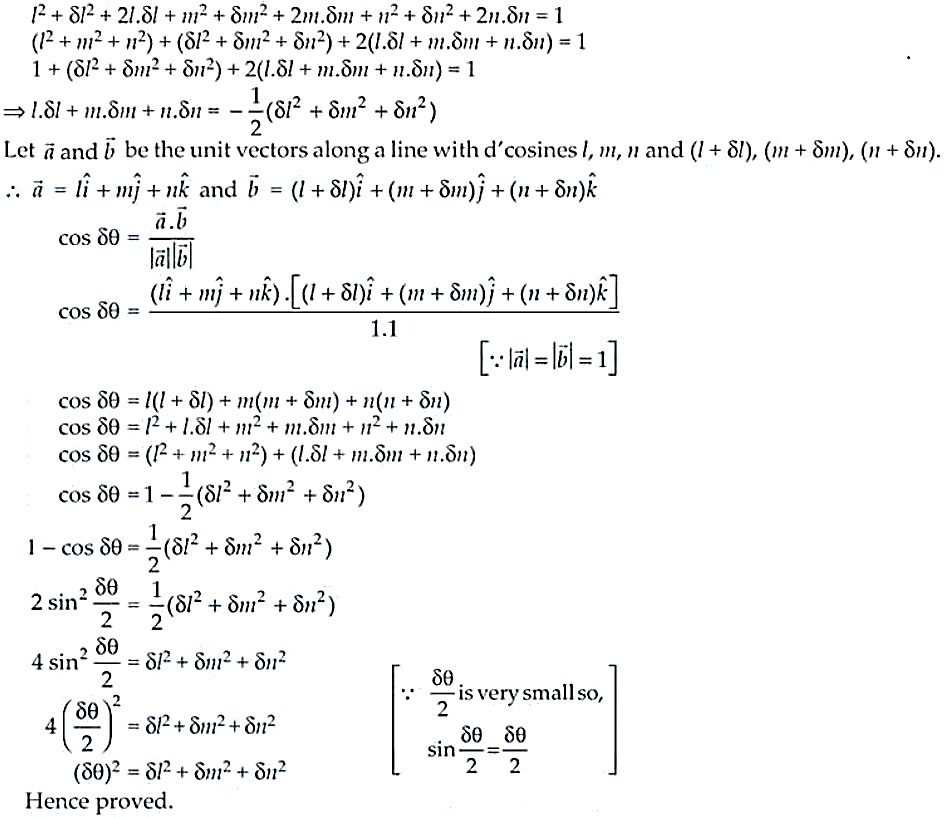14. O is the origin and A is (a, b, c). Find the direction cosines of the line OA and the equation of plane through A at right angle to OA.

Solution:

Given, O (0, 0, 0) and A(a, b, c)

So, the direction ratios of OA = a – 0, b – 0, c – 0 = a, b, c

And, the direction cosines of line OA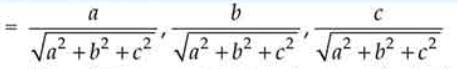Now, the direction ratios of the normal to the plane are (a, b, c).

We know that, the equation of the plan passing through the point A(a, b, c) is

a(x – a) + b(y – b) + c(z – c) = 0

ax – a2 + by – b2 + cz – c2 = 0

ax + by + cz = a2 + b2 + c2

Thus, the required equation of the plane is ax + by + cz = a2 + b2 + c2

15. Two systems of rectangular axis have the same origin. If a plane cuts them at distances a, b, c and a¢, b¢, c¢, respectively, from the origin, prove that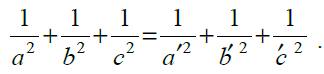Solution:

Let’s take OX, OY, OZ and ox, oy, oz to be two rectangular systems.

And, the equations of two planes are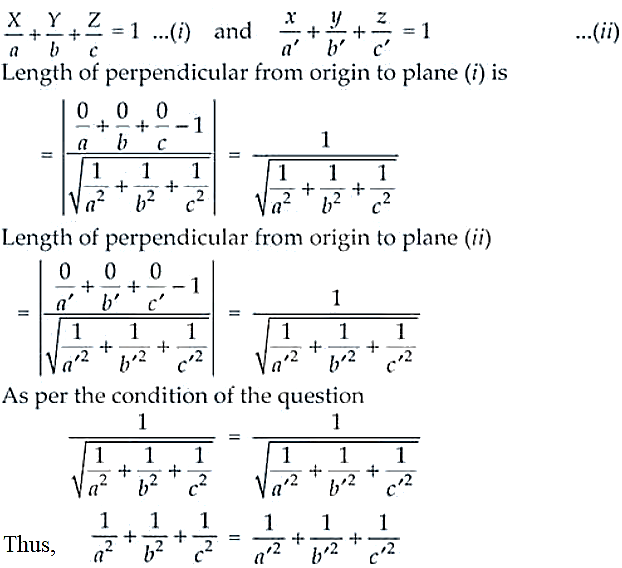16. Find the foot of perpendicular from the point (2, 3, –8) to the line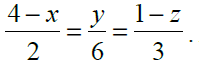Also, find the perpendicular distance from the given point to the line.

Solution:

Given,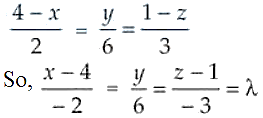is the equation of the line.

Now, the coordinates of any point Q on the line are

x = -2λ + 4, y = 6λ and z = -3λ + 1 and the given point is P(2, 3, -8)

The direction ratios of PQ are -2λ + 4 – 2, 6λ – 3, -3λ + 1 + 8 i.e. -2λ + 2, 6λ – 3, -3λ + 9

And the direction ratios of the given line are -2, 6, -3.

If PQ ⊥ line, then

-2(-2λ + 2) + 6(6λ – 3) – 3(-3λ + 9) = 0

4λ – 4 + 36λ – 18 + 9λ – 27 = 0

49λ – 49 = 0

λ = 1

Now, the foot of the perpendicular is -2(1) + 4, 6(1), -3(1) + 1 i.e. 2, 6, -2

Hence, the distance PQ is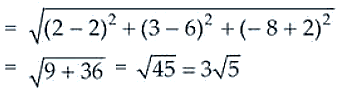Thus, the required coordinates of the foot of perpendicular are 2, 6, -2 and the required distance is 3√5 units.

17. Find the distance of a point (2, 4, –1) from the line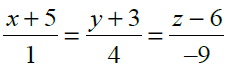Solution: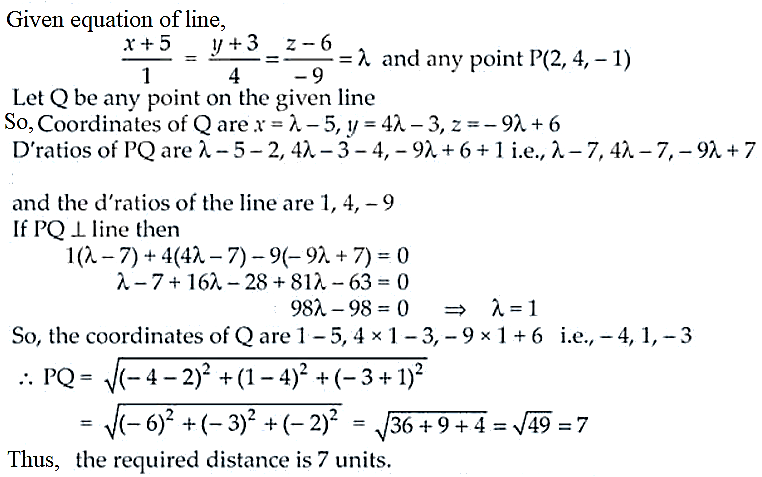18. Find the length and the foot of perpendicular from the point (1, 3/2, 2) to the plane 2x – 2y + 4z + 5 = 0.

Solution:

Given plane is 2x – 2y + 4z + 5 = 0 and point (1, 3/2, 2)

The direction ratios of the normal to the plane are 2, -2, 4

So, the equation of the line passing through (1, 3/2, 2) and direction ratios are equal to the direction ratios of the normal to the plane i.e. 2, -2, 4 is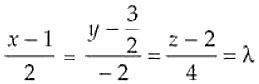Now, any point in the plane is 2λ + 1, -2λ + 3/2, 4λ + 2

Since, the point lies in the plane, then

2(2λ + 1) – 2(-2λ + 3/2) + 4(4λ + 2) + 5 = 0

4λ + 2 + 4λ – 3 + 16λ + 8 + 5 = 0

24λ + 12 = 0 λ = ½

So, the coordinates of the point in the plane are

2(-1/2) + 1, -2(-1/2) + 3/2, 4(-1/2) + 2 = 0, 5/2, 0

Thus, the foot of the perpendicular is (0, 5/2, 0) and the required length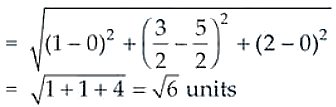19. Find the equations of the line passing through the point (3,0,1) and parallel to the planes x + 2y = 0 and 3y z = 0.

Solution:

Given point is (3, 0, 1) and the equation of planes are

x + 2y = 0 …. (i) and 3y – z = 0 …. (ii)

Equation of any line l passing through (3, 0, 1) is

l: (x – 3)/a = (y – 0)/b = (z – 1)/c

Now, the direction ratios of the normal to plane (i) and plane (ii) are (1, 2, 0) and (0, 3, 1).

As the line is parallel to both the planes, we have

1.a + 2.b + 0.c = 0 ⇒ a + 2b + 0c = 0 and

0.a + 3.b – 1.c = 0 ⇒ 0a + 3b – c = 0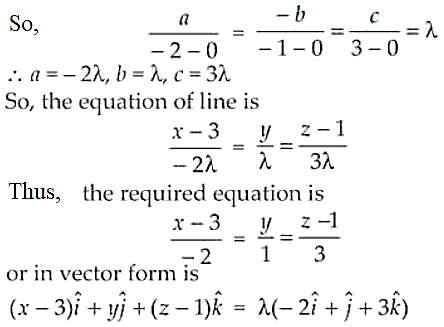20. Find the equation of the plane through the points (2, 1, –1) and (–1, 3, 4) and perpendicular to the plane x – 2y + 4z = 10.

Solution:

We know that, equation of the plane passing through two points (x1, y1, z1) and (x2, y2, z2) with its normal’s direction ratios is

a(x – x1) + b(y – y1) + c(z – z1) = 0 …. (i)

Now, if the plane is passing through two points (2, 1, -1) and (-1, 3, 4) then

a(x2 -x1) + b(y2 – y1) + c(z2 – z1) = 0

a(-1 – 2) + b(3 – 1) + c(4 + 1) = 0

-3a + 2b + 5c = 0 …. (ii)

As the required plane is perpendicular to the given plane x – 2y + 4z = 10, then

1.a – 2.b + 4.c = 10 …. (iii)

On solving (ii) and (iii) we get,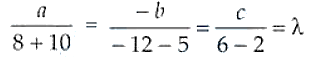So, a = 18λ, b = 17λ and c = 4λ

Thus, the required plane is

18λ (x – 2) + 17λ (y – 1) + 4λ (z + 1) = 0

18x – 36 + 17y – 17 + 4z + 4 = 0

⇒ 18x + 17y + 4z – 49 = 0

21. Find the shortest distance between the lines given by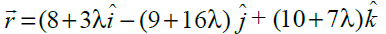and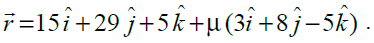Solution:

Given equations of lines are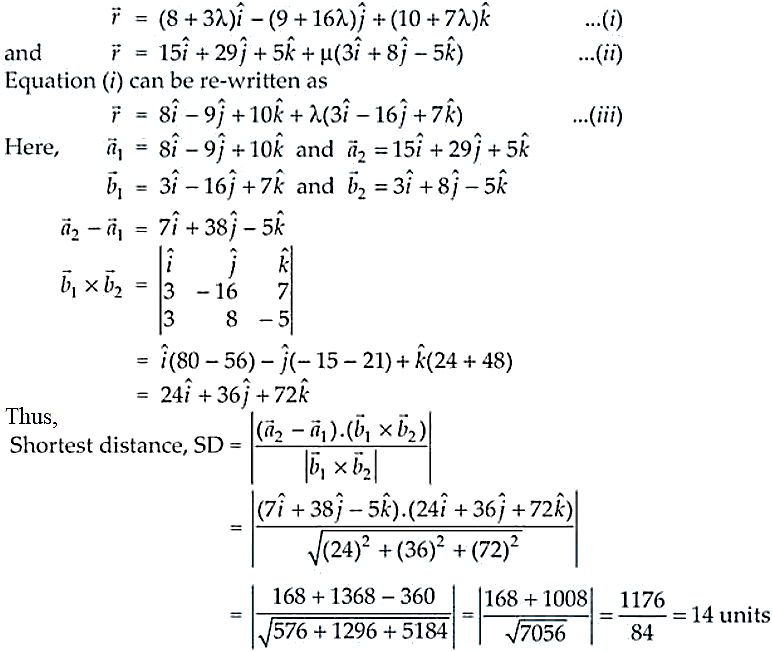22. Find the equation of the plane which is perpendicular to the plane 5x + 3y + 6z + 8 = 0 and which contains the line of intersection of the planes x + 2y + 3z – 4 = 0 and 2x + y z + 5 = 0.

Solution:

The given planes are

P1: 5x + 3y + 6z + 8 = 0

P2: x + 2y + 3z – 4 = 0

P3: 2x + y – z + 5 = 0

Now, the equation of the plane passing through the line of intersection of P1 and P3 is

(x + 2y + 3z – 4) + λ(2x + y – z + 5) = 0

(1 + 2λ)x + (2 + λ)y + (3 – λ)z – 4 + 5λ = 0 …. (i)

From the question its understood that plane (i) is perpendicular to P1, then

5(1 + 2λ) + 3(2 + λ) + 6(3 – λ) = 0

5 + 10λ + 6 + 3λ + 18 – 6λ = 0

7λ + 29 = 0

λ = -29/7

Putting the value of ; in equation (i), we get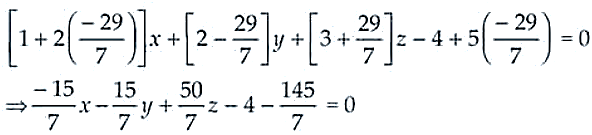-15x – 15y + 50z – 28 – 145 = 0

-15x – 15y + 50z – 173 = 0 ⇒ 51x + 15y – 50z + 173 = 0

Thus, the required equation of plane is 51x + 15y – 50z + 173 = 0.

23. The plane ax + by = 0 is rotated about its line of intersection with the plane z = 0 through an angle α. Prove that the equation of the plane in its new position is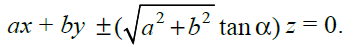Solution:

Given planes are: ax + by = 0 …. (i) and z = 0 …. (ii)

Now, the equation of any plan passing through the line of intersection of plane (i) and (ii) is

(ax + by) + kz = 0 ⇒ ax + by + kz = 0 …. (iii)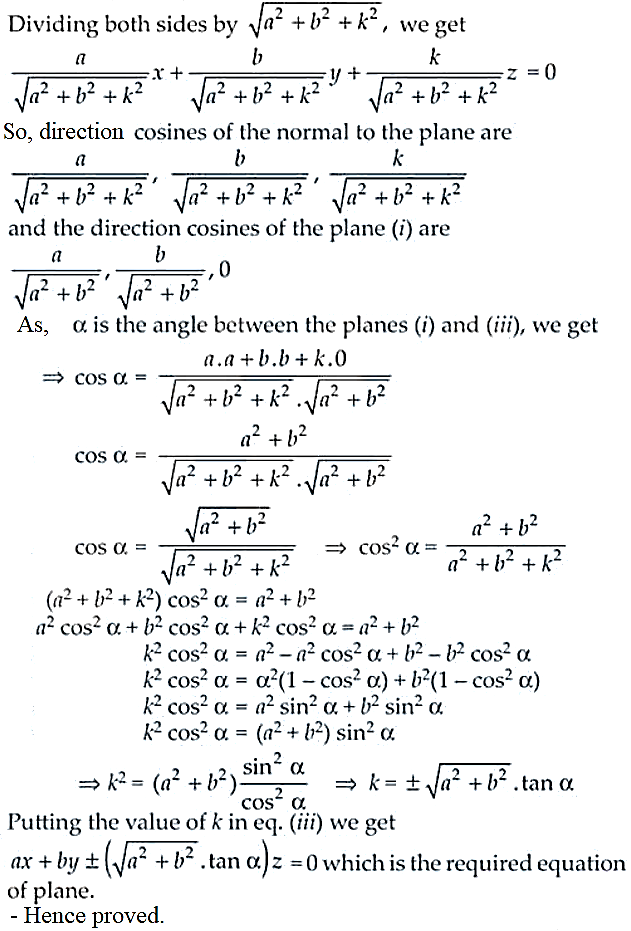24. Find the equation of the plane through the intersection of the planes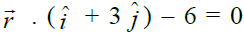and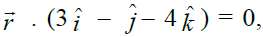whose perpendicular distance from origin is unity.

Solution: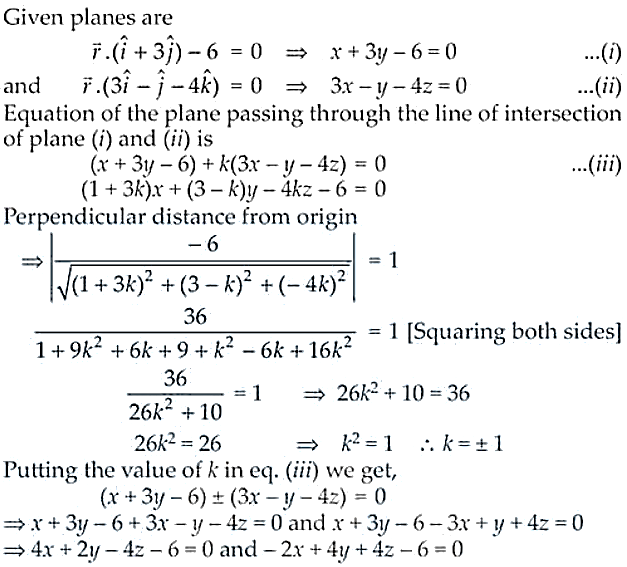Thus, the required equations of planes are

4x + 2y – 4z – 6 = 0 and -2x – 4y + 4z – 6 = 0.